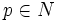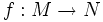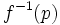# Regular value theorem

Let$M, N$ be differential manifolds and$p \in N$ be a regular value of a differentiable map$f: M \to N$. Then$f^{-1}(p)$ is a submanifold of$M$.
A slightly stronger version of this result states the following: if there is an open neighbourhood$U$ of$p$ in$N$ such that the rank of the Jacobian is constant for all points in$f^{-1}(U)$, then$f^{-1}(p)$ is a submanifold of$M$.